Home / Blog / Data Science / Lambda Function in Python

# Lambda Function in Python

• June 27, 2023
• 6437
• 41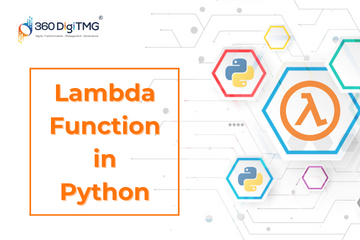### Meet the Author : Mr. Bharani Kumar

Bharani Kumar Depuru is a well known IT personality from Hyderabad. He is the Founder and Director of Innodatatics Pvt Ltd and 360DigiTMG. Bharani Kumar is an IIT and ISB alumni with more than 17 years of experience, he held prominent positions in the IT elites like HSBC, ITC Infotech, Infosys, and Deloitte. He is a prevalent IT consultant specializing in Industrial Revolution 4.0 implementation, Data Analytics practice setup, Artificial Intelligence, Big Data Analytics, Industrial IoT, Business Intelligence and Business Management. Bharani Kumar is also the chief trainer at 360DigiTMG with more than Ten years of experience and has been making the IT transition journey easy for his students. 360DigiTMG is at the forefront of delivering quality education, thereby bridging the gap between academia and industry.

Alonzo Church introduced the lambda function to the field of mathematics in the 1930s. Python has an anonymous function called a lambda that is used to define functions without a reputation. In contrast to regular functions, lambda functions evaluate a function, accept any number of arguments, and return a single expression.

The lambda function has no name, in contrast to the 'def' function. It is a very straightforward Python function that just requires the lambda keyword, parameters, and expressions in a single line of code.

### Syntax of Lambda Functions:

variable = lambda parameters_list: expression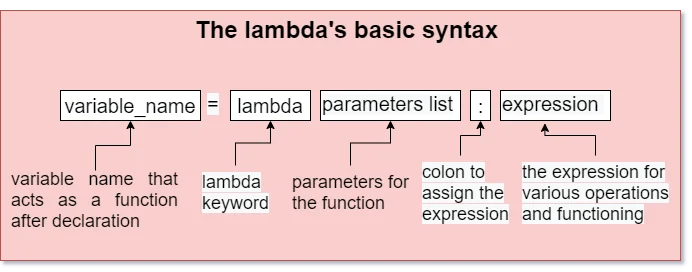### Parts of Lambda Function:

• Lambda Keyword
• Parameters(variables)
• Function body

### Characteristics of lambda functions:

• The lambda function can have any number of arguments but only one expression, which is evaluated and returned.
• Lambda functions are often used wherever function objects are required.
• The lambda functions are syntactically restricted only to one expression.
• It is written in a single line of code.
• Anonymous functions may be used inside another function.
• They need not require a return statement.
• These functions are used for a short period.
• It's simple and usable inside another function.

### Normal Function Vs Lambda Function:

NORMAL FUNCTION LAMBDA FUNCTION
Def keyword is employed to define the function, together with its function name Lambda keyword is employed to define the function.
Return statements are defined to return the values. Return statements do not seem to be required.
In this case, it returns an integer, In this case, it returns the function object
The execution time is slow The execution time is fast
More lines of code are written and hence it takes a longer time. One line of code is written and hence it takes less time.

### Operations in Lambda Function

The following operations can be carried out with the help of the lambda function;

• Subtraction
• Multiplication
• Division

• Using functional programming
• Using object-oriented programming
• Parameterizing a method of a particular class

### Let's see with a few examples:

add = lambda a, b: a + b
# output: 10

Explanation: A lambda object add is defined first. The lambda expression is then initialised with the two arguments a and b. These two parameters are numbers that will be added together. The definition of the addition phrase follows the colon.

• Subtraction

subtract = lambda a, b: a - b
# output: 150

Explanation: A lambda object subtract is declared first. The lambda expression is then initialised with the two arguments a and b. The subtraction operation is to be carried out on these two parameters, which are integers. The definition of the subtraction expression follows the colon.

• Multiplication

multiply = lambda a, b: a * b
print(multiply(100, 50))
# output: 5000

Explanation: A lambda object multiply is declared first. The lambda expression is then initialised with the two arguments a and b. These two parameters are numbers that will be multiplied together. After the colon, we define the multiplication expression.

• Division

div = lambda a, b: a / b
print(div(100, 50))
# output: 2

Explanation: A lambda object div is declared first. The lambda expression is then initialised with the two arguments a and b. These two parameters are numbers that will be used to divide. The definition of the division phrase follows the colon.

### Lambda functions with if-else conditions:

Lambda expressions with conditional statements are a very useful technique and also the lines of code can be reduced. In this, the lambdas can hold only one expression at a time

What is the syntax when we use lambda with if-else conditions in Python:

variable_name = lambda parameters: code_for_if if (condition) else code_for_else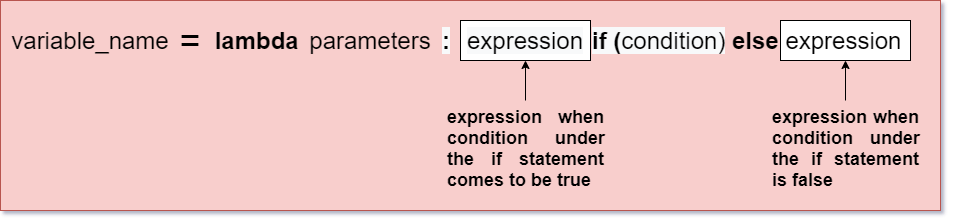In this syntax we define the expression before the if statement and then write the if statement with the condition, and the else block is defined after the if condition.

conditional_lambda = lambda x: x/100 if x < 20 else x
print(conditional_lambda(5))
# output: 0.05

Explanation:

• First, create a lambda object as conditional_lambda.
• Next, define the lambda keyword with the argument x and pass the one-line expression.
• The condition says that if x is less than 20 divide it by 100 else print it as it is.
• Then we call the conditional_lambda function and inside it, we give the parameter as 5.
• As 5 is less than 20 it will get divided by 100 and the output is displayed as 0.05.

### Let’s discuss some advanced methods of using Lambda Functions:

Example 1: Check whether the given number is an even or odd using the lambda function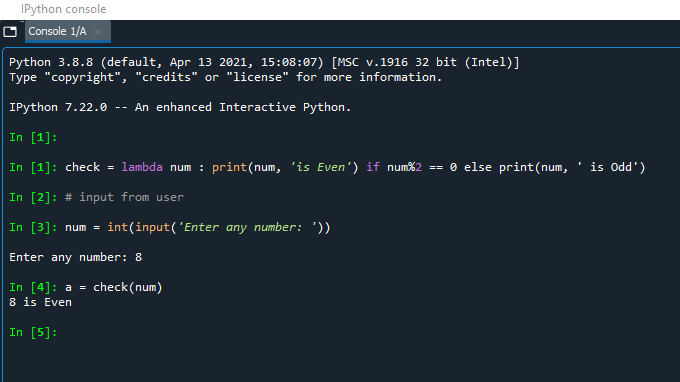Explanation:

• First, the variable name check is defined, and the keyword lambda is defined with parameter num.
• Then after the print condition if statement is created. The main condition is the parameter num if divided by 2 returns zero, If the condition is satisfied and it prints(“num is even”).
• But if the remainder is not zero then the statement inside the else block is printed(“num is odd”).
• Since we entered the value as 8, it is exactly divided by 2 and the output is an even number.

Example 2: Check whether the given number is divisible or not by another number by getting two inputs from the user using the lambda function.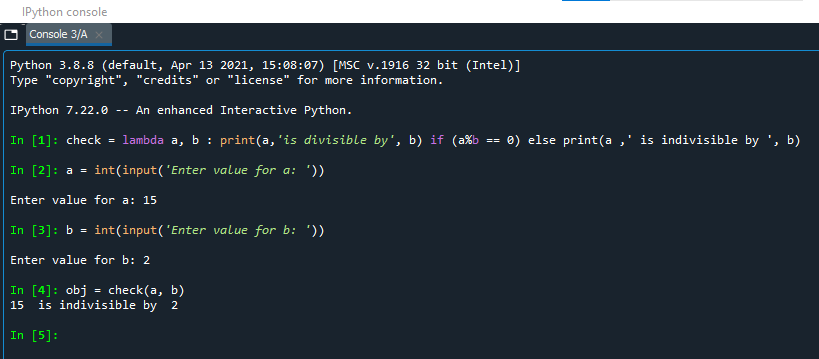Explanation:

• First, the keyword lambda is defined with the two arguments a and b. Next, the variable name check is defined.
• Then following the creation of the if statement. The primary requirement is that the parameter a be divisible by b. If the condition is met, the sentence "a is divisible" is printed. However, the statement included in the else block is written if the remainder is not zero.
• Then we create a function object as obj and call the check function passing the parameters inside(a, b).
• Since we entered the value as 15 for a and 2 for b, it is not exactly divisible. Hence the statement inside the else block is printed.

### Can we reuse the lambda function?

We can reuse the lambda function to add one number to the existing numbers. Let’s look into a simple example,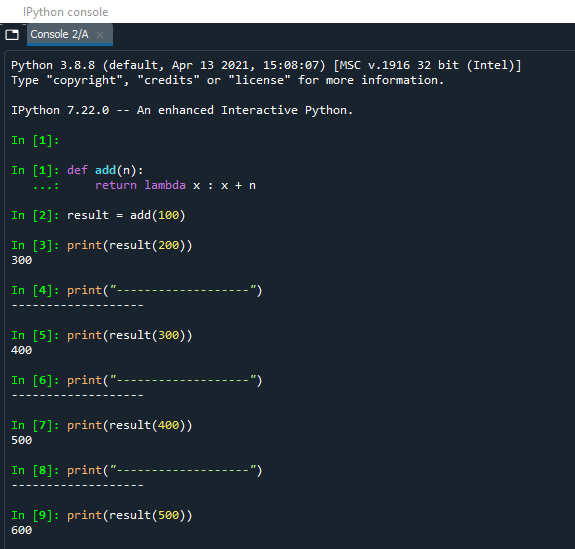### Uses of Lambda Function:

• We can give a name and use it as a normal function.
• It can be used together with higher-order functions like map(), filter(), and reduce().
• Lambda functions can be used to reduce the lines of code.
• Lambda functions assign to key arguments which gives more flexibility.
• It can be used to make precise programs, especially in the case of nested functions.
• Lambda function can be immediately invoked.

### Lambda functions in python DataFrames:

A. We will now explore how to use lambda functions on a single column using dataframe.assign() method.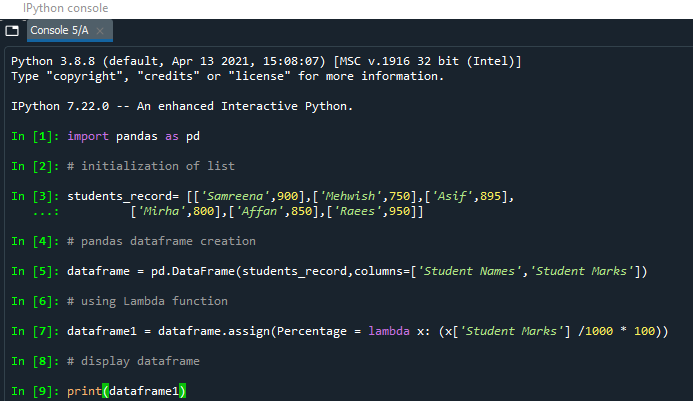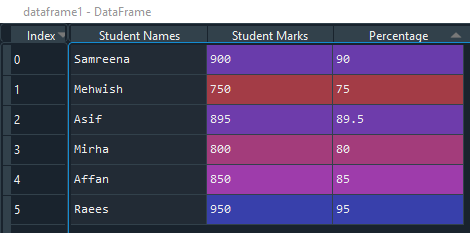Explanation:

• First import pandas package for data manipulation.
• Next, initialize the list of students with their marks.
• Create a pandas data frame with student names and marks as columns.
• Lambda function is applied to the column student marks.
• After the lambda function is applied, the student percentages are calculated and stored in a new column - percentage.

B. We will now explore how to use lambda functions on multiple columns using dataframe.assign() method.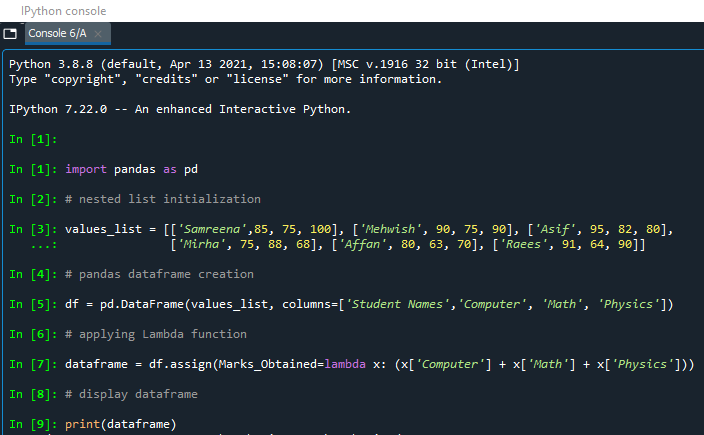Explanation:

• First import pandas package for data manipulation.
• Next, initialize the list of students with their marks for three subjects.
• Create a pandas data frame with student names, computer, maths, and physics as columns.
• Lambda function is applied to the column computer, math, and physics to calculate the marks obtained for each student.
• Those marks are stored in the new column marks_obtained, in the data frame.

C. We will now explore how to use lambda functions on a single row using dataframe.apply() method.Explanation:

• First import pandas package for data manipulation.
• Create a pandas data frame with id, names, age, and a monthly income as columns.
• The Lambda function is applied to the monthly income column to increment the amount by 1000 for each person.

D. We will now explore how to filter the data with lambda functions using filter() method.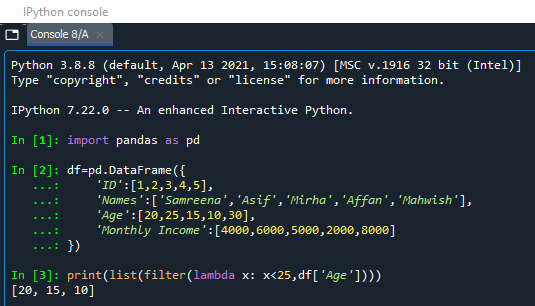Explanation:

• First import pandas package for data manipulation.
• Create a pandas data frame with id, names, age, and a monthly income as columns.
• Lambda function is applied to the age column to filter the age of the people under 25 years.

E. We will now explore how to map the data with lambda functions using map() method.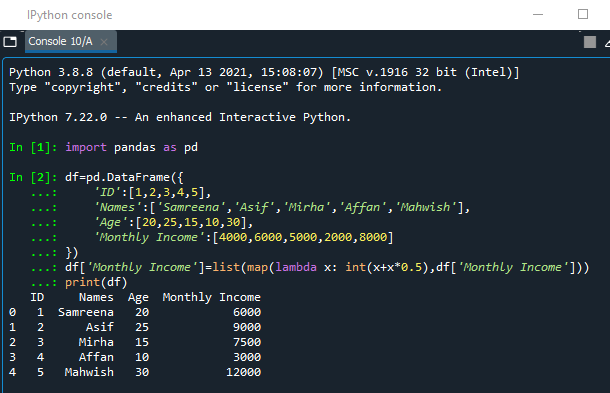Explanation:

• First import pandas package for data manipulation.
• Create a pandas data frame with id, names, age, and a monthly income as columns.
• Lambda function is applied to the monthly income column to substitute or replace a series with other values.

### Data Analyst Courses in Other Locations